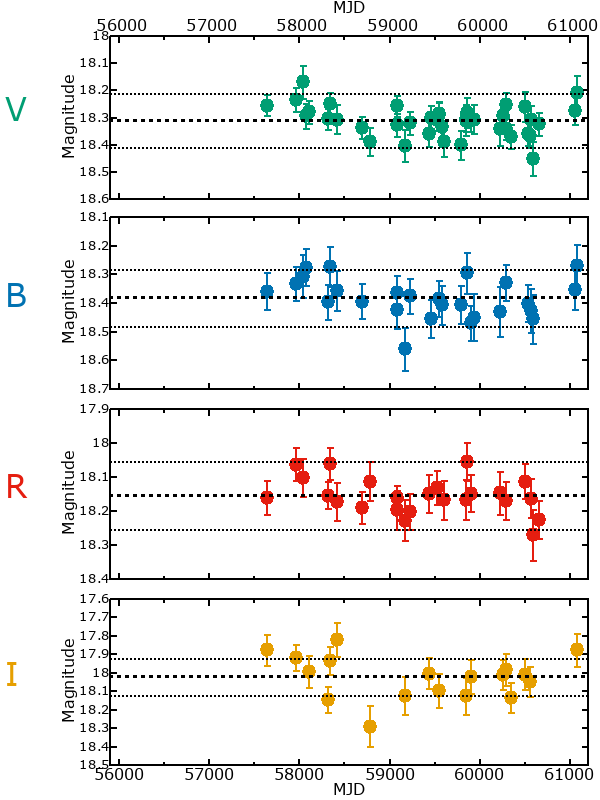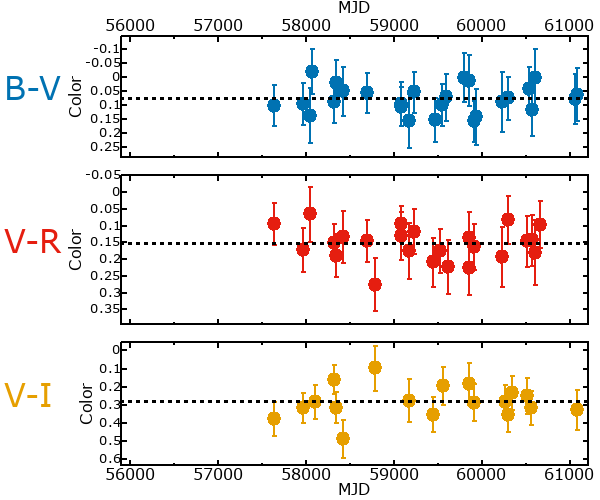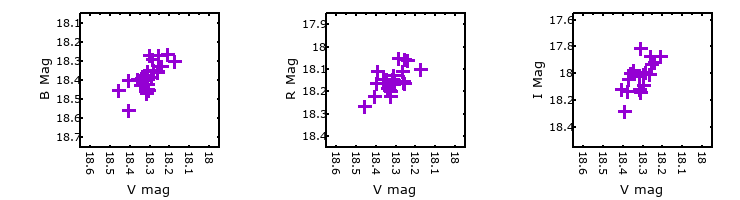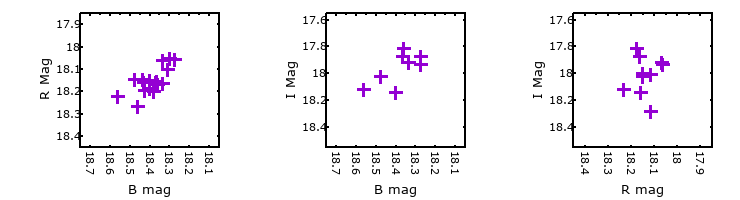#M33-013446.93 (J013446.93+305426.5)

## Plots of Brightness and Color## Variability

It is likely that this star is variable.

### Correlation factors between bands

If the brightness variation is true variability then there should be a correlation between brightness changes recorded in different filter bands. The following table are the R-squared linear correlation factors calculated for the pairs of bands. The number in parentheses next to the R-squared value is the number of elements used to calculate the correlation factor. The number in square brackets next to the band is the range of magnitudes measured.

V [0.29 mag]B [0.25 mag]R [0.16 mag]
B [0.29 mag]0.67 (12)
R [0.16 mag]0.41 (12)0.60 (11)
I [0.47 mag]0.55 (8)0.47 (6)0.06 (7)

### Magnitude-Magnitude Plots

The plots below are provided to check the values in the table above and also to show any non-linear correlations### I & R Statistics

Welch & Stetson (1993, AJ, 105, 1813) present a method for varible star detection in CCD images from automated surveys. Data from two bands are compared and two statistics are calculated: R and I.

The I-statistic has an expectation value of zero for stars that are NOT variable. A larger non-zero I-value indicates a higher likelihood that the star is variable. The R statistic indicates how the color of the star changes as the variable grows brighter with R = 1.0 being no change in color.

R and I values have only been calculated if there are more than four (4) matched measurements of brightness in each band.
V
(I|R)
B
(I|R)
R
(I|R)
B
(I|R)
0.95 | 0.77
R
(I|R)
0.84 | 1.281.02 | 0.15
I
(I|R)
1.50 | 0.661.32 | 0.660.69 | 0.55